# 15 Converter Instructions in Siemens PLC Programming | Free PLC Tutorials

The programmable logic controller has several converter instructions. Let’s study converter instructions in the Siemens PLC programming.

Till now you can see the various instructions of the PLC like Logic Gates, Bit Logic Instructions, 20+ Arithmetic instructions, PLC Timers and PLC Counters, etc.

There are 15 converter instructions you can find while programming. Let’s study each of these instructions in detail.

## Converter Instructions in PLC Programming

### Integer to BCD conversionThis instruction converts the integer value into a three-digit Binary Coded Decimal value. It can convert integer value into BCD up to +/- 999.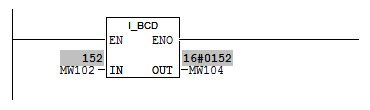### Double Integer to BCD Conversion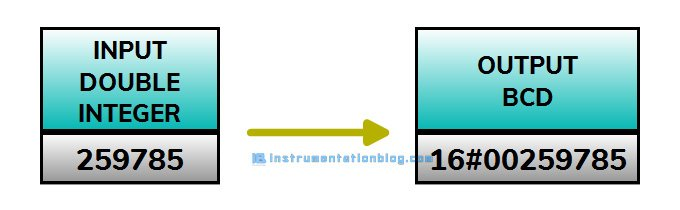This instruction converts the double integer value into a seven-digit Binary Coded Decimal value. It can convert double integer value into BCD up to +/- 9999999.### BCD to Integer ConversionThis instruction converts a three-digit Binary Coded Decimal value into an integer value.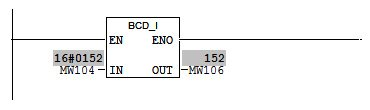### BCD to Double Integer ConversionThis instruction converts a seven-digit Binary Coded Decimal value into a double integer value.### Integer to Double Integer Conversion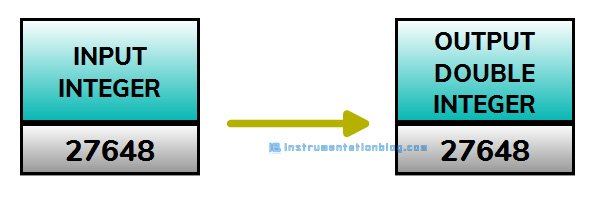This instruction converts an integer value(16-bit) in double integer value(32-bit). The input parameter is a single word while the output parameter is the double word here.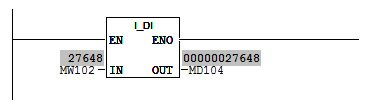### Double Integer to Real ConversionThis instruction converts a double integer value into a floating-point number.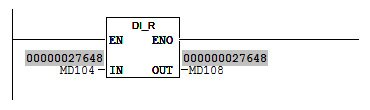### Invert Integer(One’s Complement of Integer)This instruction does the one’s complement with the 16-bit integer value and gives the output. Here both the input and output values are 16-bit.### Invert Double Integer(One’s Complement of Double Integer)This instruction does the one’s complement with the 32-bit integer value and gives the output. Here both the input and output values are 32-bit.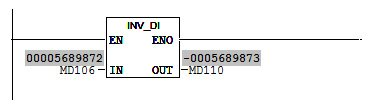### Negative Integer(Two’s Complement of Integer)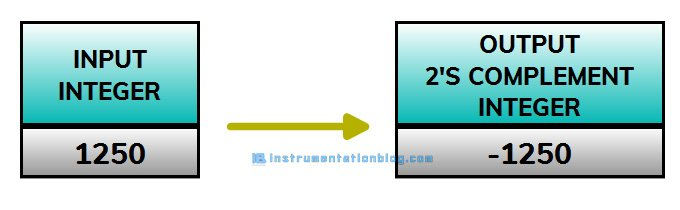This instruction does the two’s complement with the 16-bit integer value and gives the output. Here both the input and output values are 16-bit.### Negative Double Integer(Two’s Complement of Double Integer)This instruction does the two’s complement with the 32-bit integer value and gives the output. Here both the input and output values are 32-bit.### Negative RealThis instruction multiplies the input floating-point number by -1 and gives the output. The input and output both here are floating-point numbers.### ROUNDThis instruction reads the input value as a floating-point number and converts it into a 32-bit double integer value. The result is the nearest integer number.### TRUNCThis instruction reads the input value as a floating-point number and converts it into a 32-bit double integer value. The result is the nearest integer number to zero.### CEILThis instruction reads the input value as a floating-point number and converts it into a 32-bit double integer value. The result is the nearest integer number to the + infinity.### FLOORThis instruction reads the input value as a floating-point number and converts it into a 32-bit double integer value. The result is the nearest integer number to the – infinity.### Summary

These are all the converter instructions in PLC. Please feel free to give your feedback in the comment section below. You can also find my previous tutorial about the plc programming instructions.

What is PLC? How does it work?

Logic gates using PLC ladder logic.

Basic bit logic instructions with PLC programming examples.

5 different types of PLC programming languages.

Three different types of electric motor starter with its PLC program.

30+ Most useful PLC communication protocols

How timer works in PLC?

How counter works in PLC?

You can also find the important questions and answers asked in the interview.

25+ Most important questions asked in the PLC interview